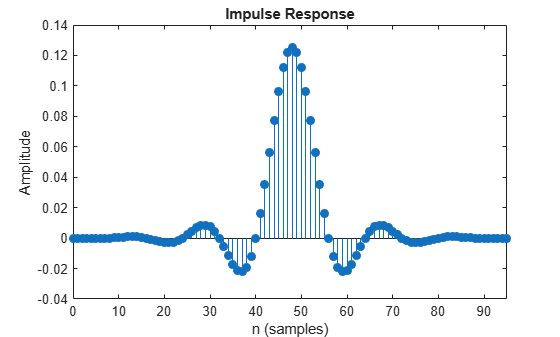# coeffs

Coefficients of prototype lowpass filter

## Syntax

``c = coeffs(obj)``

## Description

example

````c = coeffs(obj)` returns the coefficients of the prototype lowpass filter in `dsp.Channelizer` and `dsp.ChannelSynthesizer` System objects.```

## Examples

collapse all

Determine the coefficients of the prototype lowpass filter in the `dsp.Channelizer` object using the `coeffs` function.

The `coeffs` function returns a structure, c. The structure field `Numerator` contains the coefficients as a row vector. The number of elements in the row vector, given by `c.Numerator`, equals the number of frequency bands times the number of coefficients per band. These values are given by the `NumFrequencyBands` and `NumTapsPerBand` properties of the `dsp.Channelizer` object.

```channelizer = dsp.Channelizer; c = coeffs(channelizer);```

Visualize the impulse response of the filter using `fvtool`.

`fvtool(c.Numerator,'impulse');`## Input Arguments

collapse all

Input filter, specified as either a `dsp.Channelizer` or a `dsp.ChannelSynthesizer` System object.

Example: channelizer = dsp.Channelizer;

Example: ```channelizer = dsp.ChannelSynthesizer```

## Output Arguments

collapse all

Lowpass filter coefficients, returned as a structure. The structure field `Numerator` contains the coefficients as a row vector.

## Version History

Introduced in R2016b